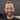# Connecting the Dots of Monte Carlo

February 20, 2021

Monte Carlo ad nauseum. Predicting diseases from known genes. Estimating genes from observed diseases. Blatant abuse of function notation.

✽✽✽

I know I just discussed Monte Carlo (MC) simulations, but there’s so much to unpack with these things. In this conversation, I want to take a step back and explore MC techniques while avoiding the distracting details. No code. Limited equations.

## Monte Carlo Simulations—Going Forward

A simulation is a function that maps a set of parameters to an output:

$(y_1, y_2, \dots, y_m) = f_\text{sim}(\theta_1, \theta_2, \dots, \theta_n)$

Practically, this could be a simulation that takes $n$ binary values representing the presence or absence of a gene and produces a set of $m$ characteristics representing a possible expression of those genes. The interaction between genes could be very complex. However, if we run lots of simulations we can see what expressions typically occur for a particular combination of genes. For example, if genes $\theta_1$ and $\theta_2$ are present, how often does disease $y_1$ occur across any state of the remaining $\theta_3, \dots, \theta_n$ genes?

Monte Carlo simulations inform us about the outcomes/observations if we know the parameters/hidden states that describe the generating process.

## Monte Carlo Inference—Going Backward

In many situations, we may have the simulation model, $f_\text{sim}$, but we do not know the parameters $\theta_i$. Knowing the parameters would tell us valuable information about the process, so we want to estimate them from observations of many outcomes.

One simple way to do this would be to pick random values for each $\theta_i$, run the simulation, and compare the results to all our observations. We randomly keep parameter sets based on how closely their simulation outputs match the observation data. After many random samples of parameters, we can average each parameter to get its expected value given our observations. This is a bit like trying to find a needle in a haystack, however, and more parameters in the simulation means a bigger haystack. For more than a couple parameters, it will take a long time to get enough accepted parameter guesses to confidently estimate the expected values. The spread or variance among the samples will tell use how confident we can be in the mean value. If the samples are clustered tightly together, we can be more confident than if the samples were evenly distributed.

Markov chain Monte Carlo (MCMC) solves this problem by positing that “good” sets of parameters will occur next to each other. Instead of generating a every random sample of the parameters from scratch, MCMC takes the last set of accepted parameters and tweaks it slightly. After running a simulation and comparing the output to the our observations, we accept the parameters if they ended up being better than the old ones. If they produced worse output, we might accept them with some probability based on how bad they were. Thus, we start with a random sample and then stochastically “walk” around the probability distribution until we find enough good samples to compute the expected value (by taking the mean).

MCMC assumes we treat the observations as a single batch. Sequential Monte Carlo techniques can efficiently generate parameter samples as observations are sequentially collected. Successive observations could be noisy GPS sensor readings, and the parameter to be estimated might be your true position.1 Particle filtering is a popular example of this approach.

Returning to the gene example, if we know that a person has disease $y_1$, MCMC can tell us what gene combination of $\theta_1$ and $\theta_2$ we can expect this person to have, and how confident can we be in that estimate.

Markov chain Monte Carlo (MCMC) informs us about the parameters/hidden states that control the generating process if we know the outcomes/observations.

## One More Time

To use Monte Carlo techniques to answer your question, first identify your simulation model, which should take parameters and produce an output. If you know the parameters that control the process, use Monte Carlo simulations to learn about the output. If you have observations of outcomes and need to discover the parameters behind them, use MCMC, particle filtering, or other parameter inference techniques.

In summary, given $\textbf y = f_\text{sim}(\boldsymbol{\theta})$:

\begin{aligned} \text{Monte Carlo Simulations} &: \boldsymbol{\theta} \mapsto \textbf{y} &\text{(easy)}\\ \text{MCMC/PF/Inference} &: \textbf{y} \mapsto \boldsymbol{\theta} &\text{(hard)} \end{aligned}

1. In this example, the simulation takes a true position and maps it to a noisy sensor reading based on the measurement distribution.Written by Matthew Russell who follows Jesus, studies machine learning at the University of Kentucky, and interns at NASA. Get to know him or check out his projects on GitHub.